# Exploratory analysis II

Data visualization, part 2. Code for Quiz 8.

1. Load the R package we will use.
``````library(tidyverse)
library(patchwork)
``````
1. Quiz questions
• Replace all the ???s. These are answers on your moodle quiz.
• Run all the individual code chunks to make sure the answers in this file correspond with your quiz answers
• After you check all your code chunks run then you can knit it. It won’t knit until the ??? are replaced
• The quiz assumes that you have watched the videos, downloaded (to your examples folder) and worked through the exercises in exercises_slides-50-61.Rmd
1. Pick one of your plots to save as your preview plot. Use the ggsave command at the end of the chunk of the plot that you want to preview.

# Question: modify slide 51

• Create a plot with the ‘mpg’ dataset
• assign the variable ‘displ’ to the x-axis
• assign the variable ‘hwy’ to the y-axis
• add ‘facet_wrap’ to split the data into panels based on the manufacturer
``````ggplot(data = mpg) +
geom_point(aes(x = displ, y = hwy)) +
facet_wrap(facets = vars(manufacturer))
``````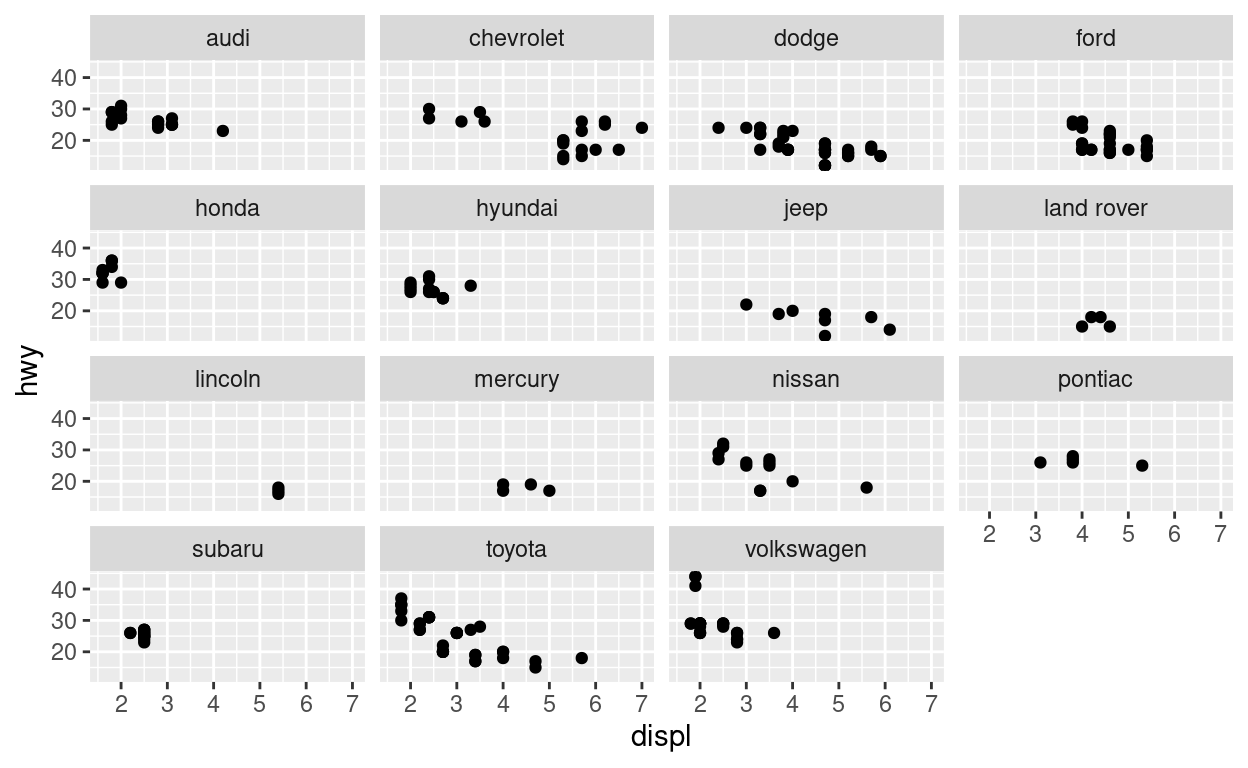# Question: modify facet-ex-2

• Create a plot with the ‘mpg’ dataset
• add bars with with ‘geom_bar’
• assign the variable ‘manufacturer’ to the y-axis
• add ‘facet_grid’ to split the data into panels based on the ‘class’
• let scales vary across columns
• let space taken up by panels vary by columns
``````ggplot(mpg) +
geom_bar(aes(y = manufacturer)) +
facet_grid(vars(class), scales = "free_y", space = "free_y")
``````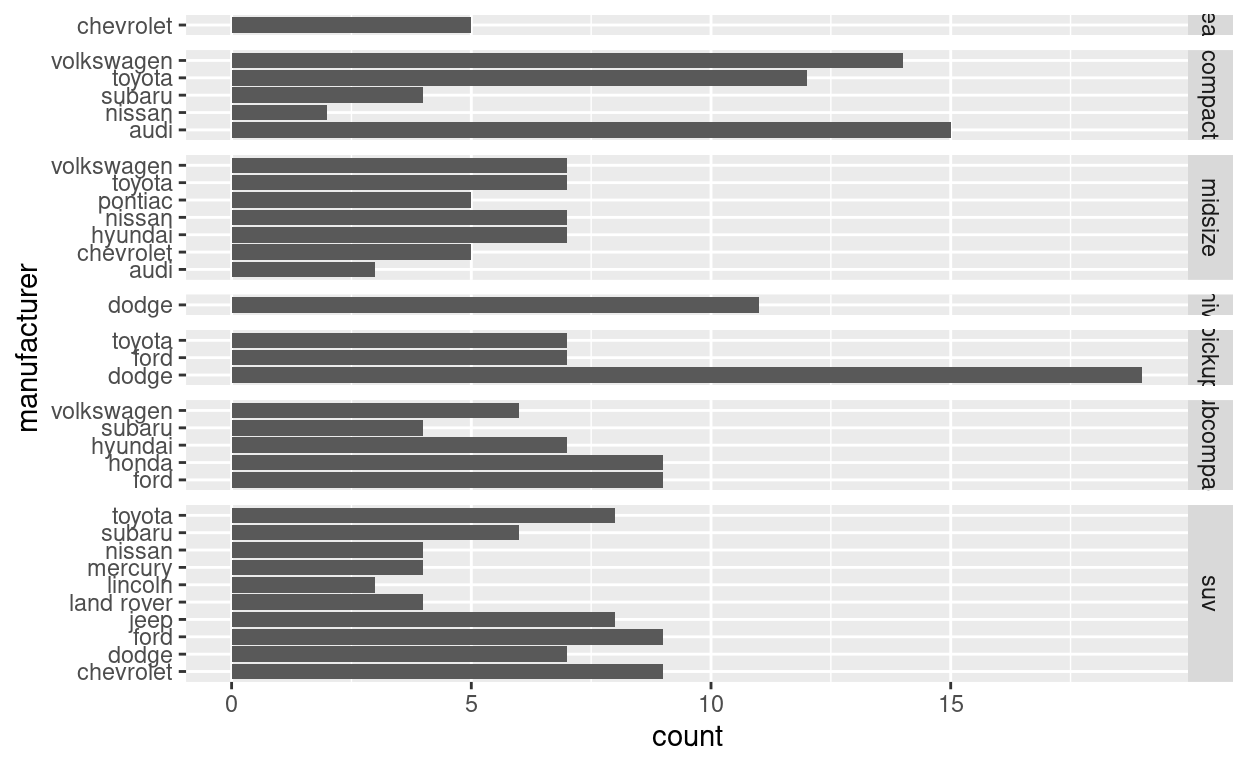# Question: spend_time

``````spend_time  <- read_csv("https://estanny.com/static/week8/spend_time.csv")
``````

• extract observations for 2011
• THEN create a plot with that data
• ADD a barchart with with ‘geom_col’
• assign ‘activity’ to the x-axis
• assign ‘avg_hours’ to the y-axis
• assign ‘activity’ to fill
• ADD ‘scale_y_continuous’ with breaks every hour from 0 to 6 hours
• set ‘subtitle’ to Avg hours per day: 2011
• set ‘x’ and ‘y’ to NULL so they won’t be labeled
• assign the output to ‘p1’
• display ‘p1’
``````p1  <- spend_time %>% filter(year == "2011")  %>%
ggplot() +
geom_col(aes(x = activity, y = avg_hours, fill = activity)) +
scale_y_continuous(breaks = seq(0, 6, by = 1)) +
labs(subtitle = "Avg hours per day: 2011", x = NULL, y = NULL)

p1
``````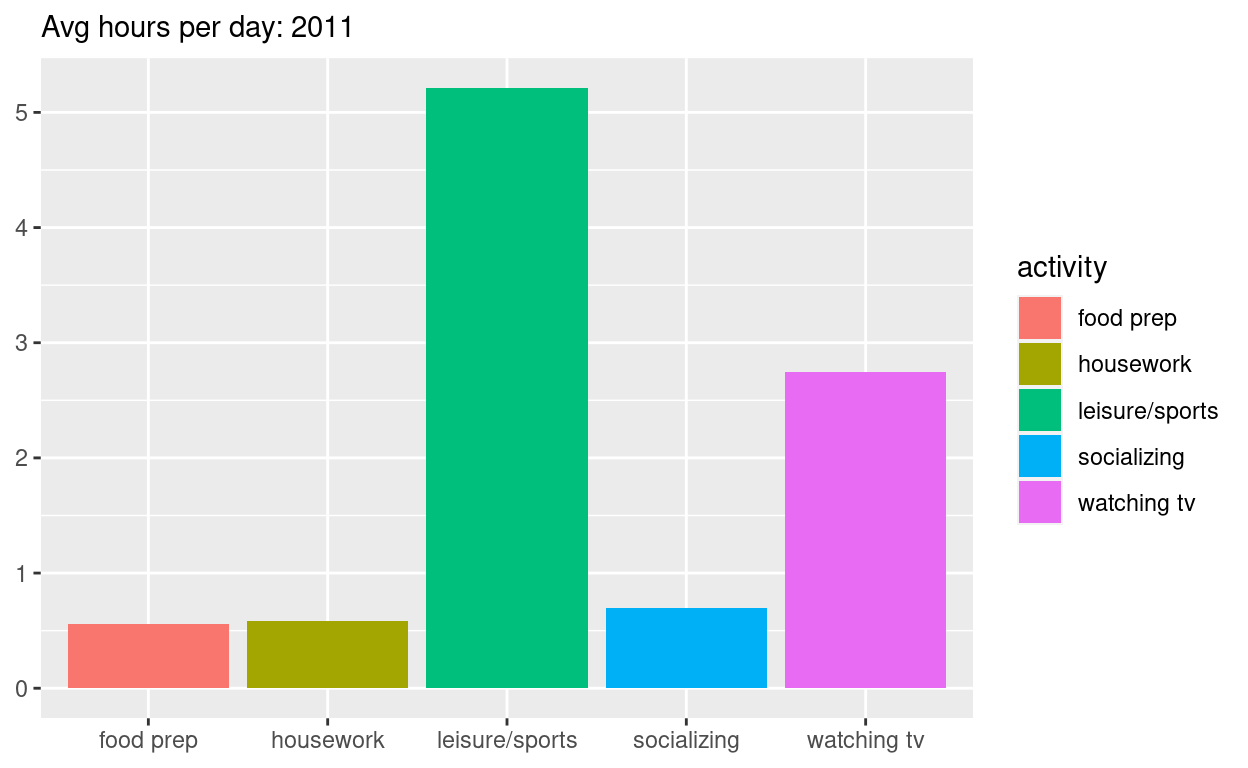• THEN create a plot with it
• ADD a barchart with with ‘geom_col’
• assign ‘year’ to the x-axis
• assign ‘avg_hours’ to the y-axis
• assign ‘activity’ to fill
• set subtitle to “Avg hours per day: 2010-2019”
• set x and y to NULL so they won’t be labeled
• assign the output to ‘p2’
• display ‘p2’
``````p2  <- spend_time  %>%
ggplot() +
geom_col(aes(x = year, y = avg_hours, fill = activity)) +
labs(subtitle  = "Avg hours per day: 2010-2019", x = NULL, y = NULL)

p2
``````Use patchwork to display ‘p1’ on top of ‘p2’

• assign the output to ‘p_all’
• display ‘p_all’
``````p_all  <-  p1 / p2

p_all
``````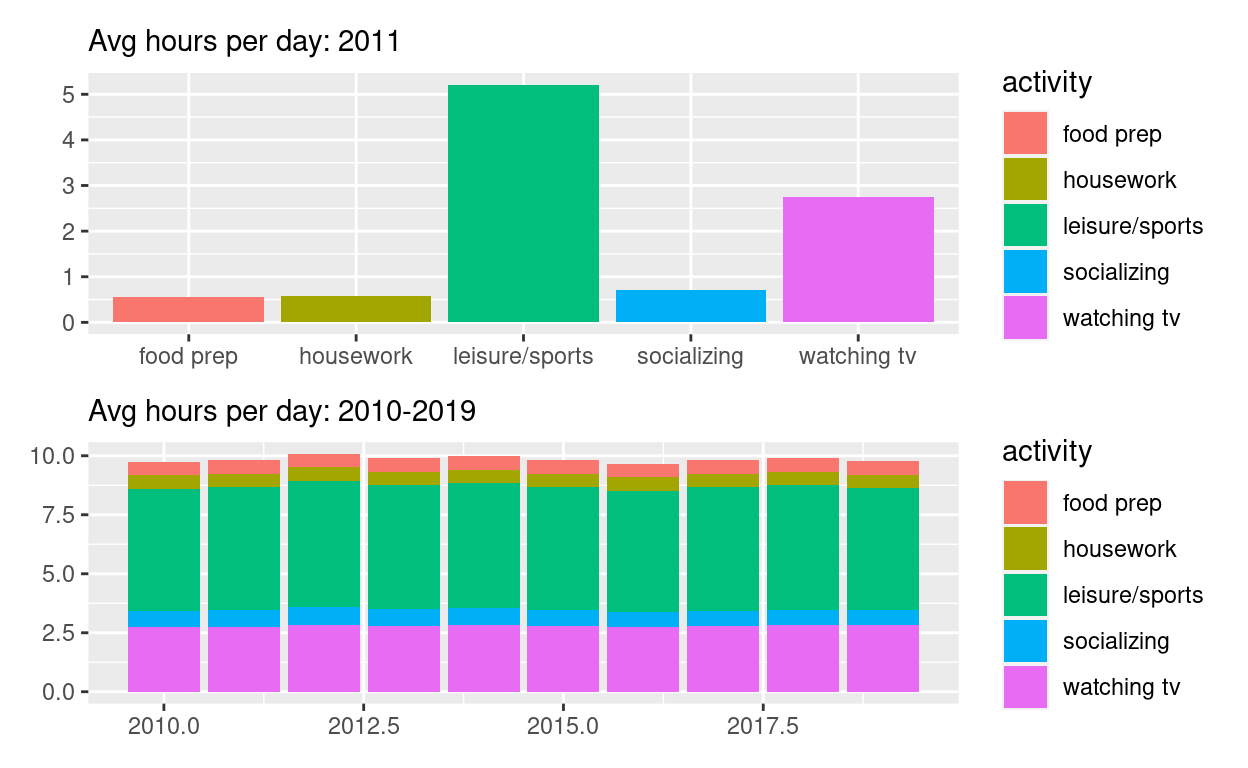• AND set ‘legend.position’ to ‘none’ to get rid of the legend
• assign the output to ‘p_all_no_legend’
• display ‘p_all_no_legend’
``````p_all_no_legend  <- p_all & theme(legend.position = 'none')
p_all_no_legend
``````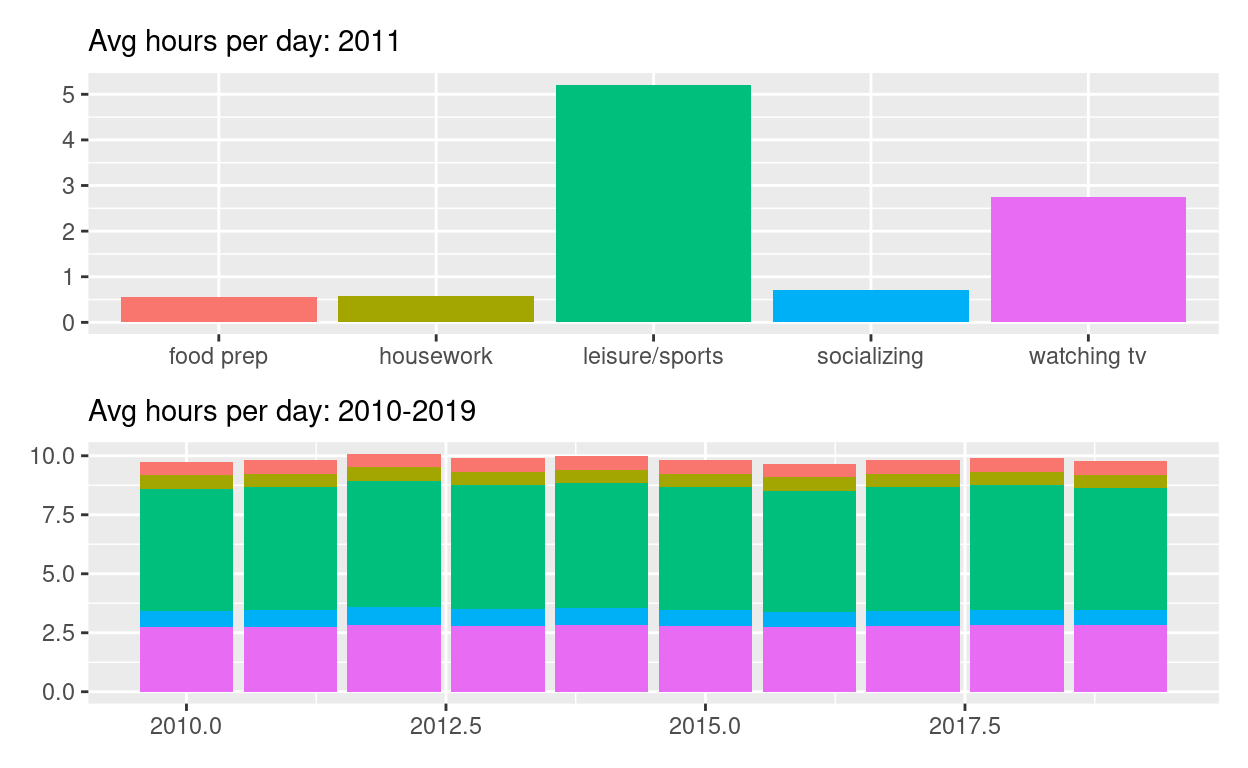``````p_all_no_legend  +
plot_annotation(title = "How much time Americans spent on selected activities",
caption = "Source: American Time of Use Survey, https://data.bls.gov/cgi-bin/surveymost?tu")
``````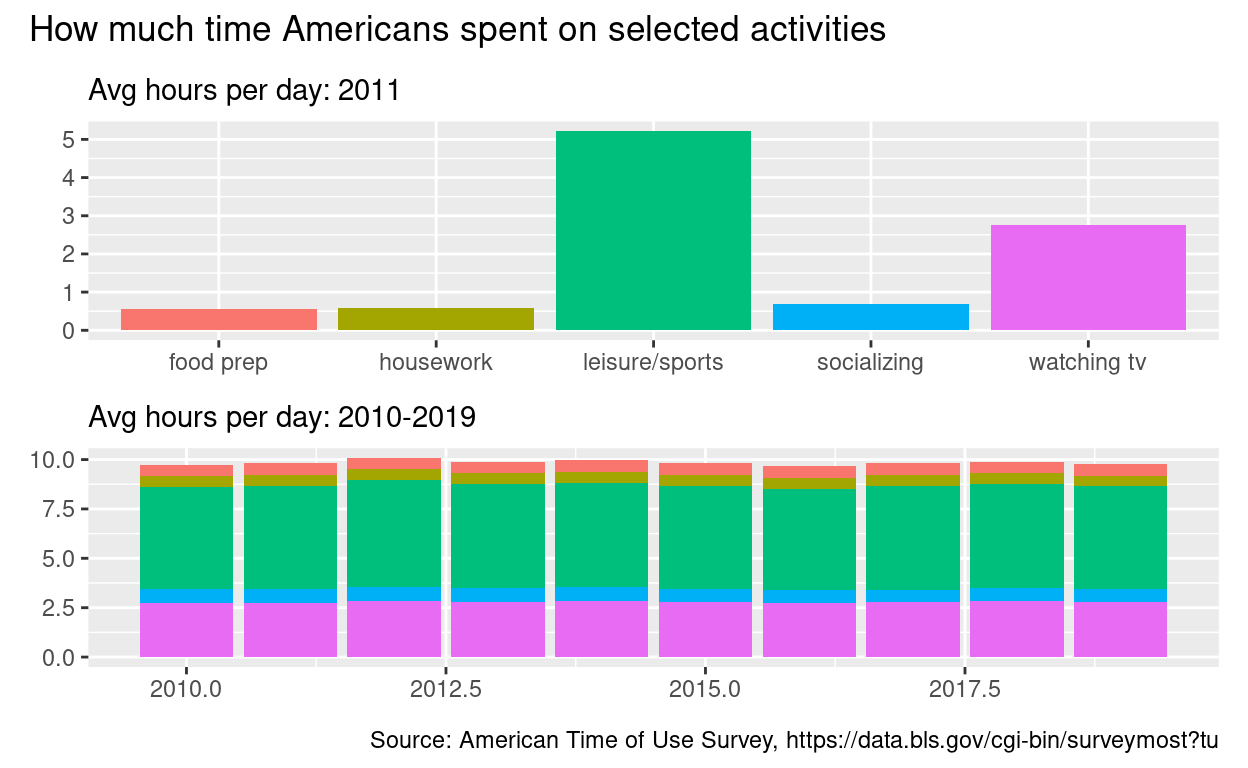# Question: Patchwork 2

use ‘spend_time’ from last question patchwork slides

• extract observations for housework
• THEN create a plot with that data
• assign ‘year’ to the x-axis
• assign ‘avg_hours’ to the y-axis
• assign ‘year’ to the x-axis
• assign ‘avg_hours’ to the y-axis
• ADD breaks on for every year on x axis with with ‘scale_x_continuous’
• set ‘subtitle’ to Avg hours per day: housework
• set ‘x’ and ‘y’ to NULL so x and y axes won’t be labeled
• assign the output to ‘p4’
• display ‘p4’
``````p4  <-
spend_time %>% filter(activity == "housework")  %>%
ggplot() +
geom_point(aes(x = year, y = avg_hours)) +
geom_smooth(aes(x = year, y = avg_hours)) +
scale_x_continuous(breaks = seq(2010, 2019, by = 1)) +
labs(subtitle = "Avg hours per day: housework", x = NULL, y = NULL)

p4
``````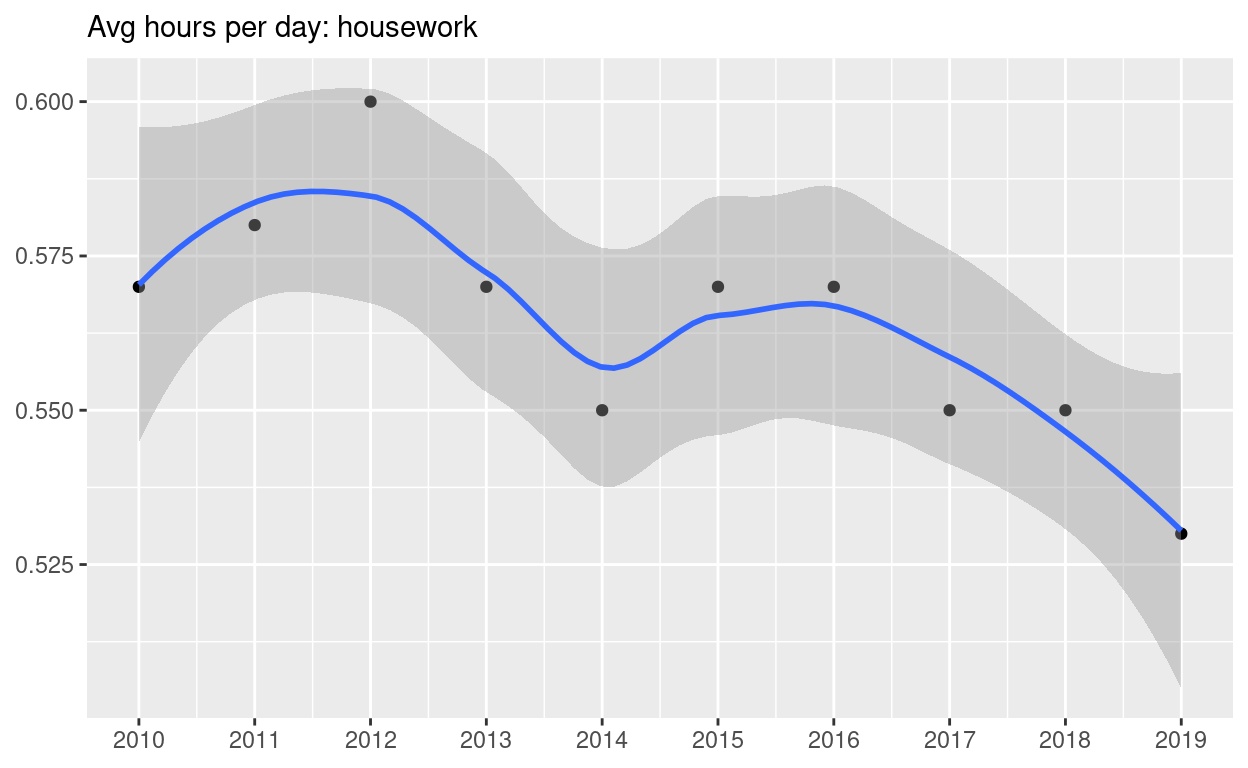• ADD ‘coord_cartesian’ to change range on y axis to 0 to 6
• assign the output to ‘p5’
• display ‘p5’
``````p5 <-  p4 + coord_cartesian(ylim = c(0, 6))
p5
``````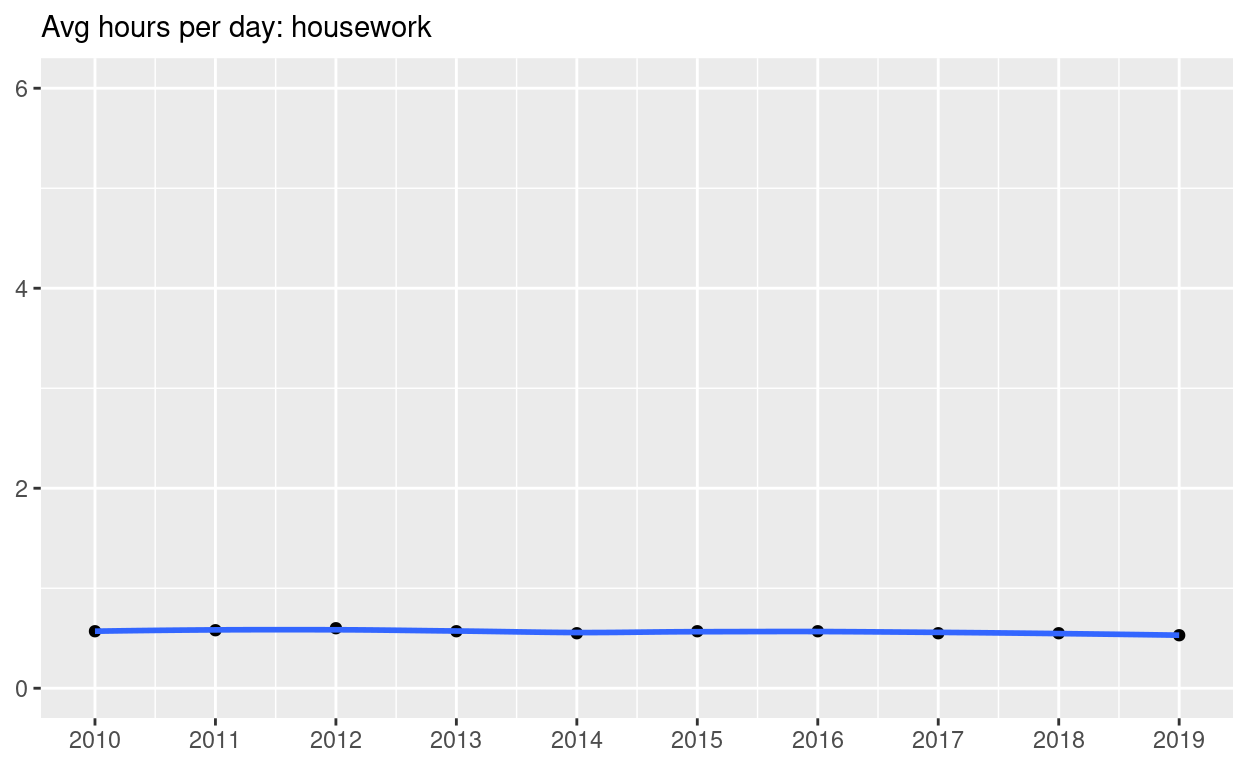• create a plot with that data
• assign ‘year’ to the x-axis
• assign ‘avg_hours’ to the y-axis
• assign ‘activity’ to color
• assign ‘activity’ to group
• assign ‘year’ to the x-axis
• assign ‘avg_hours’ to the y-axis
• assign ‘activity’ to color
• assign ‘activity’ to group
• ADD breaks on for every year on x axis with with ‘scale_x_continuous’
• ADD ‘coord_cartesian’ to change range on y axis to 0 to 6
• set ‘x’ and ‘y’ to NULL so they won’t be labeled
• assign the output to ‘p6’
• display ‘p6’
``````p6   <-
spend_time  %>%
ggplot() +
geom_point(aes(x = year, y = avg_hours, color = activity, group = activity)) +
geom_smooth(aes(x = year, y = avg_hours, color = activity, group = activity)) +
scale_x_continuous(breaks = seq(2010, 2019, by = 1)) +
coord_cartesian(ylim = c(0, 6)) +
labs(x = NULL, y = NULL)

p6
``````Use patchwork to display ‘p4’ and ‘p5’ on top of ‘p6’

``````( p4 | p5 ) / p6
``````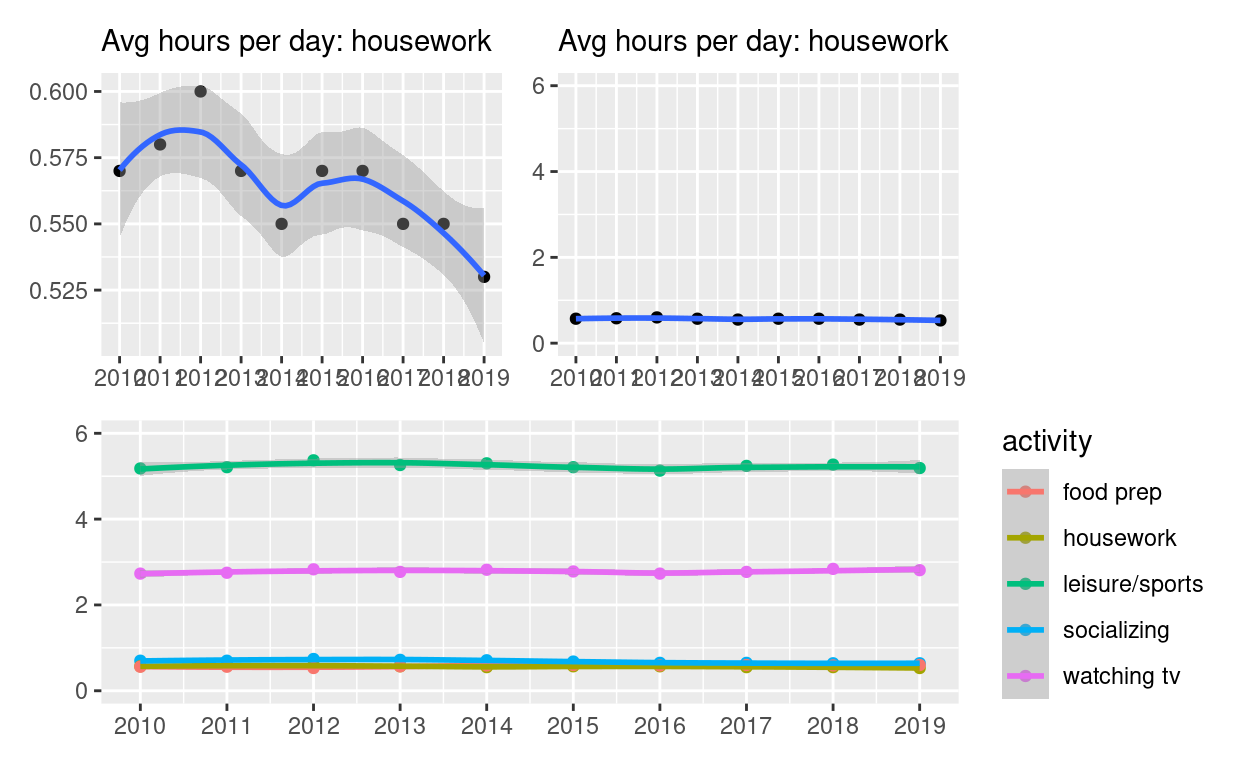``````ggsave(filename = "preview.png",
path = here::here("_posts", "2022-03-29-exploratory-analysis-ii"))
``````